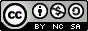Journal topic
Hydrol. Earth Syst. Sci., 1, 81–91, 1997
https://doi.org/10.5194/hess-1-81-1997Hydrol. Earth Syst. Sci., 1, 81–91, 1997
https://doi.org/10.5194/hess-1-81-199731 Mar 1997

31 Mar 1997

# A parameterization of momentum roughness length and displacement height for a wide range of canopy densities

A. Verhoef1,*, K. G. McNaughton2, and A. F. G. Jacobs1 A. Verhoef et al.
• 1Department of Meteorology, Wageningen Agricultural University, Duivendaal 2, 6701 AP, Wageningen, the Netherlands.
• 2Horticultural and Food Research Institute of New Zealand, P.O. Box 23, Kerikeri, New Zealand
• *Corresponding author. Currently at Insitute of Hydrology, Wallingford OX10 8BB, UK.

Abstract. Values of the momentum roughness length, z0, and displacement height, d, derived from wind profiles and momentum flux measurements, are selected from the literature for a variety of sparse canopies. These include savannah, tiger-bush and several row crops. A quality assessment of these data, conducted using criteria such as available fetch, height of wind speed measurement and homogeneity of the experimental site, reduced the initial total of fourteen sites to eight. These datapoints, combined with values carried forward from earlier studies on the parameterization of z0 and d, led to a maximum number of 16 and 24 datapoints available for d and z0, respectively.
The data are compared with estimates of roughness length and displacement height as predicted from a detailed drag partition model, R92 (Raupach, 1992), and a simplified version of this model, R94 (Raupach, 1994). A key parameter in these models is the roughness density or frontal area index, λ.
Both the comprehensive and the simplified model give accurate predictions of measured z0 and d values, but the optimal model coefficients are significantly different from the ones originally proposed in R92 and R94. The original model coefficients are based predominantly on measured aerodynamic parameters of relatively closed canopies and they were fitted `by eye'. In this paper, best-fit coefficients are found from a least squares minimization using the z0 and d values of selected good-quality data for sparse canopies and for the added, mainly closed canopies.
According to a statistical analysis, based on the coefficient of determination (r2), the number of observations and the number of fitted model coefficients, the simplified model, R94, is deemed to be the most appropriate for future z0 and d predictions. A CR value of 0.35 and a cd1 value of about 20 are found to be appropriate for a large range of canopies varying in density from closed to very sparse. In this case, 99% of the total variance occurring in the d-data across 16 selected canopies can be explained, whereas the analogous value for the z0-data (24 datapoints available) is 81%. This makes the R94 model, with only two coefficients and its relatively simple equations, a useful universal tool for predicting z0 and d values for all kinds of canopies.
For comparison, a similar fitting exercise is made using simple linear equations based on obstacle height only (e.g. Brutsaert, 1982) and another formula involving canopy height as well as roughness density (Lettau, 1969). The fitted Brutsaert equations explain 98% and 62% of the variance in the d and z0-data, respectively. Lettau's equation for prediction of z0 performs unsatisfactorily (r2 values <0, even after fitting of the coefficient) and so it is concluded that the drag partition model is definitely the most effective for prediction of the momentum roughness lengths for a wide rang of canopy densities.